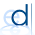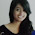## Thursday, 26 September 2013

### IMAGE PROCESSING IN VERILOG - ADD IMAGES IN VERILOG - MATLAB XILINX MODELSIM

In previous postings, we have seen how to Convert an Image into Text file for processing in HDL (verilog, VHDL) Now let us see how to process the text converted image in Verilog. We have implemented this in Xilinx and Modelsim.

The flow is simple
4. Get the sum/output
5. Write to text file.

After that using matlab function, convert the output text file back to image.

The verilog codes are as follows,,
MAIN MODULE:
input rstb,clk,en;
input [7:0] a,b;
output reg [7:0] sum;
output reg car;
always @ (negedge clk or negedge rstb) begin
if(rstb==0)
begin sum=0; car=0; end
else begin
if(en==1)
begin {car,sum}=(a+b); end //en if
end //else end
end
endmodule

TEST BENCH:
The image pixel value is read into "hexad" variable & then it is converted to decimal for processing.

//store output value @negedge since UUT is NEGEDGE sensitive ie, UUT produces output @ NegEdge
always @ (negedge clk) begin
if(rstb==1 && en==1 && w!=(n+1)) begin
if(w!=n) begin //check if count reaches max (image size)
a<=decim[w]; b<=decim2[w]; //put values for UUT
out[w]=sum; //store UUT output to array
\$fwrite(outfile,"%d\n",sum);
\$display("\n%d",sum);
w=w+1; //increment count
end
else begin a<=a; b<=b; end
end
else if(w==(n+1) && done==0) begin done=1;    \$display("Img Adding complete..."); end

This helps us to do much more better.
Thankyou.

1.1.first all gray values are read as HEXA & converted into decimal.

then for every negedge, it puts decimal_converted_val to a,b & gets the output, writes to output textfile.

The loop iterates "n" times which is the size of the image which can be changed in parameter,

2.2.this code work for hex text file. What if our file is in decimal number system. Please tell how to to write code in that case

1.first all values are read as HEXA & converted into decimal for processing...

for more check into forum.elecdude.com

3.Can u please tell me how to write this code in verilog?

4.hi, can please tell me how to write code for edge detection in verilog?

5.This was very helpful. Can you please suggest how to write a code for canny egde detection using verilog

6.Hi I am not getting the correct output
ERROR: Too many words specified in datafile y_out1.txt
ERROR: Too many words specified in datafile y_out2.txt

1.Please mail the screenshot of the error & log file, so that i can get a clear idea on the error you've obtained.

2.7.where do i get y_out and y_out2.txt files from

8.can you provide me .ucf file for the particular code?
and plz provide me the image data too..

9.is that the file should be samefor fopen and readmemh